• +91 9971497814
• info@interviewmaterial.com

# Chapter 13 Limits and Derivatives Ex-13.1 Interview Questions Answers

### Related Subjects

Question 1 : Evaluate the Given limit: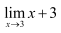Given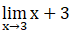Substituting x = 3, we get

= 3 + 3

= 6

Question 2 : Evaluate the Given limit: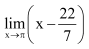Given limit:Substituting x = π, we get= (π – 22 / 7)

Question 3 : Evaluate the Given limit: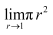Given limit:Substituting r = 1, we get= π(1)2
= π

Question 4 : Evaluate the Given limit: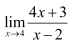Given limit:Substituting x = 4, we get= [4(4) + 3] / (4 – 2)
= (16 + 3) / 2
= 19 / 2

Question 5 : Evaluate the Given limit: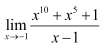Given limit:Substituting x = -1, we get= [(-1)10 + (-1)5 + 1] / (-1 – 1)
= (1 – 1 + 1) / – 2
= – 1 / 2

Question 6 : Evaluate the Given limit: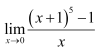Given limit:= [(0 + 1)5 – 1] / 0
=0
Since, this limit is undefined
Substitute x + 1 = y, then x = y – 1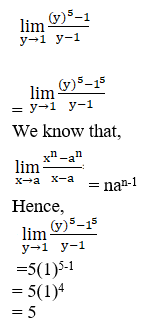Question 7 : Evaluate the Given limit: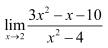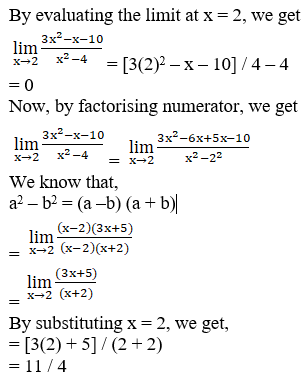Question 8 : Evaluate the Given limit: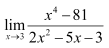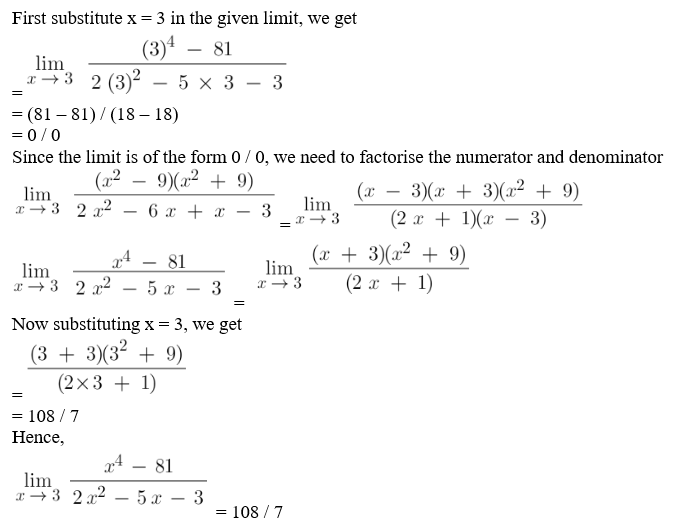Question 9 : Evaluate the Given limit: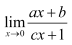Answer 9 :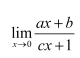= [a (0) + b] / c (0) + 1

= b / 1

= b

Question 10 : Evaluate the Given limit: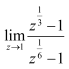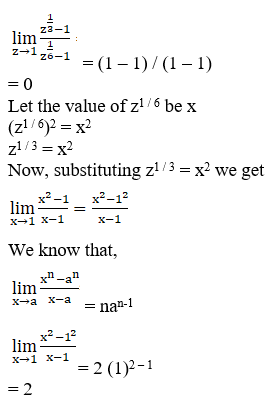Todays Deals### Chapter 13 Limits and Derivatives Ex-13.1 Contributorskrishan

Name:
Email:

# Latest News# 9000 interview questions in different categories